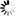DOI: 10.5176/2251-3388_2.1.28

Authors: M. Tlas

Abstract:

An interactive interior point algorithm for solving a multiobjective nonlinear programming problem has been proposed in this paper. The algorithm uses a single-objective nonlinear variant based on both logarithmic barrier function and Newton’s method in order to generate, at each iterate, interior search directions. New feasible points are found along these directions which will be later used for deriving bestapproximation to the gradient of the implicitly-known utility function at the current iterate. Using this approximate gradient, a single feasible interior direction for the implicitly-utility function could be found by solving a set of linear equations. It may be taken an interior step from the current iterate to the next one along this feasible direction. During the execution of the algorithm, a sequence of interior points will be generated. It has been proved that this sequence converges to an ε − optimal solution, whereε is a predetermined error tolerance known a priori. A numerical multiobjective example is illustrated using this algorithm

Keywords: Barrier function, Interior point method, Newton’s method, Multicriteria optimization, Multiobjective programming

Price: \$0.00Updating...